# What are three sided shapes

What is a prism
Straight prism

 ...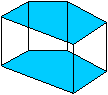... If you move any polygon along the surface normal parallel in space, a prism is created.This body is a prism in the narrower sense and is called more precisely straight prism.

Inclined prism
 ...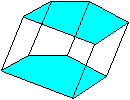... If the direction of the shift is not perpendicular to the base, a crooked prism is created.

Three-sided prism and square prism
 ...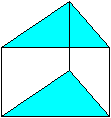... ...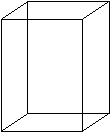... Among the prisms there are those that have triangles or squares as their base.your name is three-sided (straight) prism or square (straight) prism.

cylinder
 ...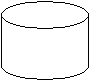... The question is whether one also counts the cylinders among the prisms.While this is not common, it can be done.

The prism used to be called a column. This term has disappeared from the mathematics textbooks.

What is a three sided prism? Top
The three-sided prism is sometimes also called a triangular prism or triangular column.

 ...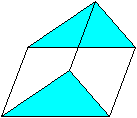... If you move any triangle parallel in space, the points in the area of ​​the displacement form a three-sided prism.It consists of two congruent triangles, the corner points of which are connected to each other. This creates three parallelograms, the side surfaces. The triangles are called base areas or, more clearly, base and top area. The distance between the triangles is the height of the prism.The term "three-sided" refers to the base, the prism is "nine-sided".

Three-sided, straight prism
 ...... If a triangle is shifted perpendicular to its surface (i.e. in the direction of the normal to the base), a straight prism is created. The sides become rectangles.The prism above is called crooked.

Regular, three-sided, straight prism
 ...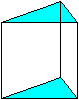... If the triangles are equilateral, the prism is also regular.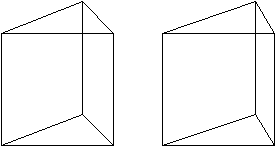Anyone who has mastered the 3D view sees a straight prism in three dimensions.

Equal-edged prism Top
This means a regular, three-sided, straight prism with edges of equal length.
presentation

 ...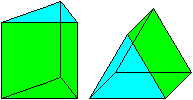... In two oblique representations (k = 1/2, alpha = 45 °) the square and on the right the equilateral triangle are shown true to the original.The prism consists of three squares and two equilateral triangles.

If you cut the body open at the edges, you get a network. There are nine different networks.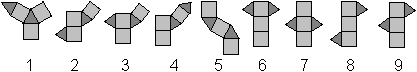Sizes
These are the side length of the triangle a, the height h = a, the volume V, the mantle M and the surface O.
 ...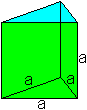... If the edge length a is given, the remaining pieces can be calculated:V = sqrt (3) / 4 * a³, M = 3 * a² and O = [sqrt (3) / 2 + 3] * a².The prism has e = 6 corners, k = 9 edges and f = 5 surfaces.Euler's polyhedron equation e + f-k = 2 applies.

Center point and sphere
 ...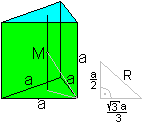... If you connect the center points of the two triangles, the center M of the prism lies in the middle of this line. This point has the same distance from all corner points of the prism. Thus he is the center of the sphere.According to the Pythagorean theorem, R = sqrt (21) / 6 * a. That is 0.76 * a

Not a ball
 ...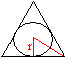... There is no such thing as a sphere that touches all 6 surfaces inside. Reason:The radius of a possible inc sphere should be r = h '/ 3 = sqrt (3) / 6 * a according to the drawing on the right. On the other hand, the prism has the height a. Then r = a / 2. That is a contradiction.

Largest regular three-sided prism Top

 ...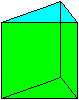... The question is what shape a regular, three-sided prism has, the volume of which is maximal and the surface of which is given.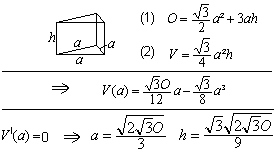Result: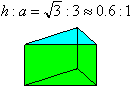It is amazing:
The same shape has a regular, three-sided prism when the surface is at its maximum for a fixed volume.

Sections through a prism Top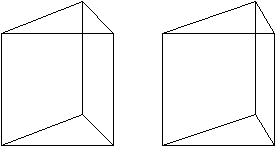You connect the two rear corner points of a prism with the opposite front corner points. Two triangles are created that mark the cutting planes through the prism. The (red) rest of the body below is a pyramid with a square as a base.
This pyramid is formed by the first cutting triangle when it is folded backwards at its center line.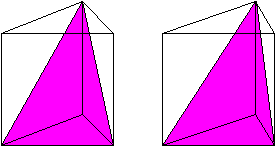If you connect the upper rear corner point with the two lower corner points, a (purple) triangle is created. This triangle is the base of a pyramid, which has its apex at the bottom at the rear corner.

If you draw a diagonal to the front of the square, you can discover two more pyramids. The special thing is that the three pyramids fill the prism and have the same volume.

Three sided prisms around us Top
When you hear the name prism, you most likely think of the glass prism with which you can split white light.

 ...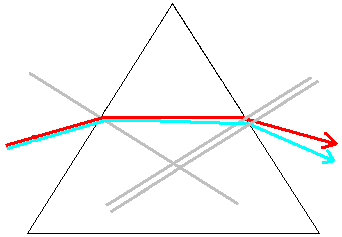... If you send a narrow bundle of white light into a prism, the light is refracted at the transition from air to glass towards the perpendicular. At the transition from glass to air, the light is deflected away from the perpendicular.What is special is that the refraction of light depends on the color. For example, blue light is refracted more strongly than red. In the drawing, the difference is exaggerated for clarity.The white light is broken down into the spectral colors.A spectrum is created.Similarly, drops of water sometimes break up white sunlight and create a rainbow.

In contrast to other bodies, the three-sided prism is not very common. The following keywords come to mind:
Ax or hatchet, BOC Tower in Hong Kong, beechnuts, Flat-Iron House in New York, kaleidoscope, wedge, gable roof, Toblerone pack, inverted prism, tent.
You can find a lot about antiprisms on my Deltaeder page.

What is a square prism? Top

 ...... A cuboid with two square sides is also called square prism.Another name is regular, four-sided prism. The old name square column has largely disappeared from the mathematics textbooks.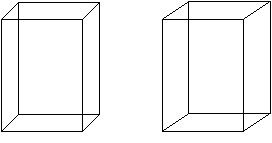Anyone who has mastered the 3D view sees the prism in three dimensions.

 ...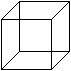The cube is also a square prism.

Sizes of the prism Top
The prism is given by the base edge a and the height h.

 ...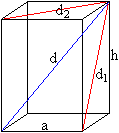... Then the lengths of the surface diagonals are d1= sqrt (a² + h²) and d2= sqrt (2) * a.The space diagonal has the length d = sqrt (i.e.1² + a²) = sqrt (2a² + h²).The surface area is O = 2a² + 4ah and the volume V = a²h.

Biggest prisms Top
Largest volume prism

 ...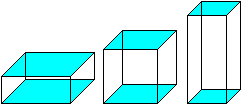... The adjacent square prisms have the same surface but different shapes.You may see that the cube in the middle has the largest volume.
This is shown by the following calculation.
Secondary condition: O = 2a² + 4ah or h = (O-2a²) / (4a)
Objective function: V = a²h or V (a) = a² (O-2a²) / (4a) = Oa / 4-a³ / 2
We have V '(a) = O / 4-3a² / 2. V '(a) = 0 gives O / 4-3a² / 2 = 0 or a = sqrt (O / 6) = sqrt (6O) / 6.
Then h = (O-2a²) / (4a) = sqrt (6O) / 6.
So a = h applies. This makes the prism a cube.

The cube can also be approached from a different direction.

Prism of the largest surface    Top
There is also the question of the prism with the largest surface area if the volume is kept constant.
Here, too, the body is a cube, as the following calculation shows.

Secondary condition: V = a²h or h = V / a² applies.
Objective function: O = 2a² + 4ah or O (a) = 2a² + 4V / a.
The following applies: O '(a) = 4a-4V / a² O' (a) = 0 results in 4a³-4V = 0 or a = V1/3 . Then h = V / a² = V / V2/3 = V1/3
So a = h applies. So here too the prism is a cube.

A box of the largest volume top
 ...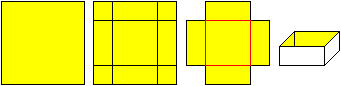... A square piece of paper is given.Remove four squares from the corners to form a cross. A box open at the top is folded out of the cross.
The question is how big the corner squares need to be so that the volume is as large as possible.
 ...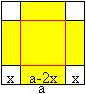... Objective function: V (x) = (a-2x) ²x = 4x³-4ax² + a²xThe first derivative is V '(x) = 12x²-8ax + a².V '(x) = 0 results in 12x²-8ax + a² = 0 or x²-2ax / 3 + a² / 12 = 0. V '' (x) = - 8a + 24x.This quadratic equation has the solutions x1= a / 6 and x2= a / 2.For x2= a / 2 there is no box. The solution is x1= a / 6 or (a-2 x1) = 2a / 3.With V '' (a / 6) = - 4a <0 it is ensured that there is a maximum.
Result: The box has the edges a / 6, 4a / 6 and 4a / 6. That is the ratio 1: 4: 4

Largest prism in the square pyramid    Top
 ...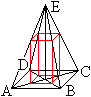... A square pyramid with the base S and the height H is given.We are looking for a square prism in the pyramid with the largest volume.For a calculation one considers a section through the points A, C and E.
invoice
 ...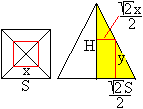... The base of the prism is x and the height is y.Then according to the second theorem of rays: [sqrt (2) S / 2] : [sqrt (2) x / 2] = H : (H-y) or y = H- (H / S) x.Objective function: V = x²y or V (x) = x² [H- (H / S) x] = Hx²- (H / S) x³V '(x) = 2Hx-3 (H / S) x². V '(x) = 0 gives 2Hx-3 (H / S) x² = 0 with the solutions x1= 0 and x2= 2S / 3.For x1= 0 there is no prism.To x2= 2S / 3 belongs to y2= H- (H / s) x2= H / 3
Result: The prism with the base side (2/3) S and the height (1/3) H has the largest volume.
If H = S, the prism is half a cube.

Two special cuboids Top

 ...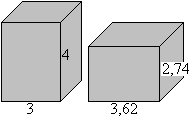... The two adjacent prisms have different bases and heights and thus different shapes, but the volumes and surfaces are the same.For the left body V = 3² * 4 = 36 and O = 2 * 3² + 4 * 3 * 4 = 66.For the right body, V = 3.62² * 2.74 = 36 and O = 2 * 3.62² + 4 * 3.62 * 2.74 = 66.However, the volume and surface are rounded to two places.The exact measurements are given below.
The following calculation shows the background.
The equation V = Oa / 4-a³ / 2 was derived above. It can also be written as a cubic equation in a: a³-Oa / 2 + 2V = 0.
The bodies are searched for for which V = 36 and O = 66.
This leads to the equation a³-33a + 72 = 0 or (a-3) (a² + 3a-24) = 0
This equation has the solutions
a1= 3, (This includes h1=4)
a2= sqrt (105) / 2-3 / 2, about 3.62. To a2 heard h2= [144 + 3sqrt (105)] / 64, roughly h2=2,73.
a3= - sqrt (105) / 2-3 / 2 <0  (No solution, a negative measure is not possible.)
This calculation also shows that just the two square prisms at the top have the volume 36 and the surface 66.

Skyscrapers and towers Top
If you pay attention, you will find that skyscrapers and towers very often have the shape of a square prism.
Sears Tower in Chicago
For skyscrapers with a square floor plan, I choose the Sears Tower in Chicago. It was through him that I came up with the idea of ​​doing this page using square prisms.
The Sears Tower consists of a bundle of 3x3 = 9 towers that are of different heights.
The skyscraper was the tallest building in the world from 1974 to 1997.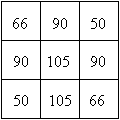The numbers indicate thatNumber of floors.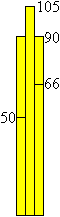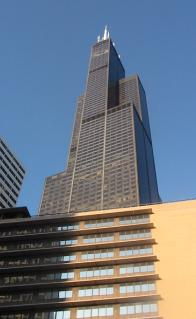September 2005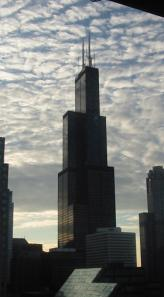September 2005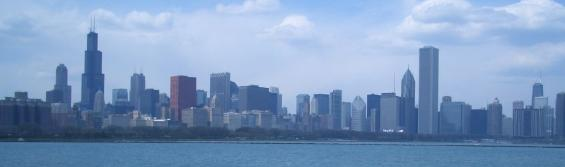Also the capital Aon center on the right is a square prism.

Now comes the leap from the cosmopolitan city to the province ;-).

For church towers, I choose the town church in Bad Salzuflen and the Kilian's church in Schötmar from my hometown.
In both cases the church towers have a square lower structure and a crown from the 19th century.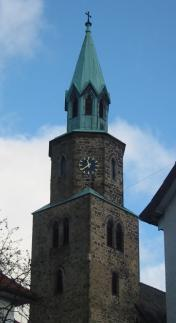The eight-pointed hoods match a square tower.

One encounters prisms in different places on my homepage.

Prism on the Internet Top

German

Wikipedia
Prism (geometry), Willis Tower

English

Ask Dr. Math (The Math Forum)
Prism Formulas

Eric W. Weisstein (MathWorld)
Prism, cuboid

John Rausch (Puzzle World)
Interlocking - Double Triangular Prism

Tom Getty
Prisms & Antiprisms

Wikipedia
Prism (geometry)

Feedback: Email address on my main page

URL of my homepage:
http://www.mathematische-basteleien.de/